### Discover more content...

Enter some keywords in the search box above, we will do our best to offer you relevant results.

### We're sorry!

We couldn't find any results for your search. Please try again with another keywords.

## 一. 什么是 Cell ？

Google S2 中定义了一个将单位球体分解成单元格层次结构的框架。每个 Cell 的单元格是由四个测地线限定的四边形。通过将立方体的六个面投影到单位球上来获得层级的顶层，通过递归地将每个单元细分为四个子层来获得较低层。例如，下面的图片显示了六个 face 中 Cell 的两个，其中一个已经细分了几次：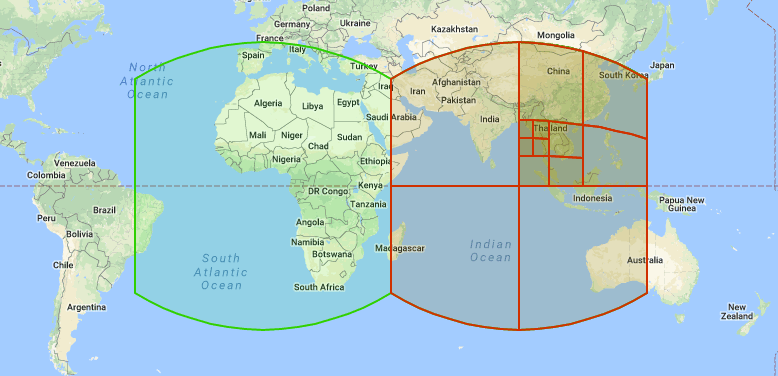S2 Level 对于空间索引和将区域逼近为单元集合非常有用。Cell 可用于表示点和区域：点通常表示为叶子节点，而区域表示为任何 Level 的 Cell 的集合。例如，下面是夏威夷近似的22个单元的集合：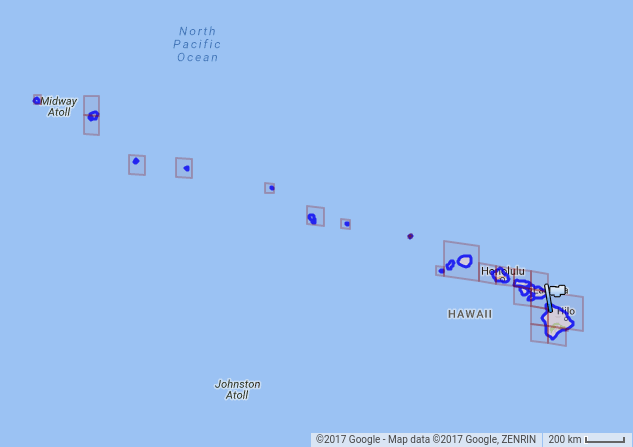## 二. S(lat,lng) -> f(x,y,z)

``````
func makeCell() {
latlng := s2.LatLngFromDegrees(30.64964508, 104.12343895)
cellID := s2.CellIDFromLatLng(latlng)
}

``````

``````
func LatLngFromDegrees(lat, lng float64) LatLng {
return LatLng{s1.Angle(lat) * s1.Degree, s1.Angle(lng) * s1.Degree}
}

``````

``````

const (
Radian Angle = 1
Degree       = (math.Pi / 180) * Radian
}

``````

LatLngFromDegrees 就是把经纬度转换成 LatLng 结构体。LatLng 结构体定义如下：

``````
type LatLng struct {
Lat, Lng s1.Angle
}

``````

``````
func CellIDFromLatLng(ll LatLng) CellID {
return cellIDFromPoint(PointFromLatLng(ll))
}

``````

``````

func PointFromLatLng(ll LatLng) Point {
cosphi := math.Cos(phi)
return Point{r3.Vector{math.Cos(theta) * cosphi, math.Sin(theta) * cosphi, math.Sin(phi)}}
}

``````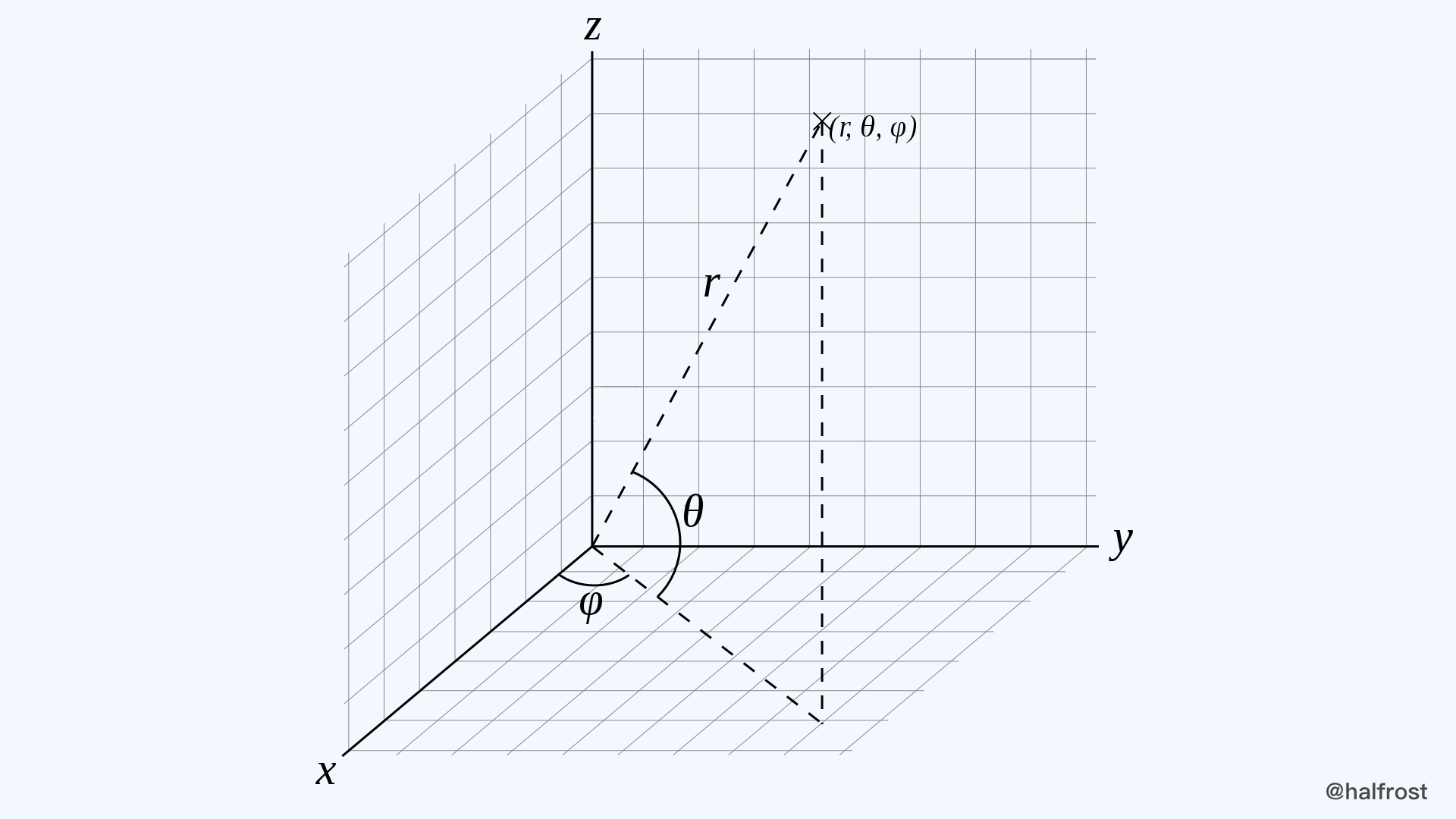θ 即为经纬度的纬度，也就是上面代码中的 phi ，φ 即为经纬度的经度，也就是上面代码的 theta 。根据三角函数就可以得到这个向量的三维坐标：

``````
x = r * cos θ * cos φ
y = r * cos θ * sin φ
z = r * sin θ

``````

``````
r3.Vector{math.Cos(theta) * cosphi, math.Sin(theta) * cosphi, math.Sin(phi)}

``````

(x, y, z) 为方向向量，它们并不要求是单位向量。(x, y, z) 的取值范围在 [-1,+1] x [-1,+1] x [-1,+1] 这样的立方体三维空间中。它们可以被标准化以使得在单位球上获得对应的点。

## 三. f(x,y,z) -> g(face,u,v)

``````
func xyzToFaceUV(r r3.Vector) (f int, u, v float64) {
f = face(r)
u, v = validFaceXYZToUV(f, r)
return f, u, v
}

``````

``````
func (v Vector) LargestComponent() Axis {
t := v.Abs()

if t.X > t.Y {
if t.X > t.Z {
return XAxis
}
return ZAxis
}
if t.Y > t.Z {
return YAxis
}
return ZAxis
}

``````

``````
const (
XAxis Axis = iota
YAxis
ZAxis
)

``````

``````

func face(r r3.Vector) int {
f := r.LargestComponent()
switch {
case f == r3.XAxis && r.X < 0:
f += 3
case f == r3.YAxis && r.Y < 0:
f += 3
case f == r3.ZAxis && r.Z < 0:
f += 3
}
return int(f)
}

``````

``````
func validFaceXYZToUV(face int, r r3.Vector) (float64, float64) {
switch face {
case 0:
return r.Y / r.X, r.Z / r.X
case 1:
return -r.X / r.Y, r.Z / r.Y
case 2:
return -r.X / r.Z, -r.Y / r.Z
case 3:
return r.Z / r.X, r.Y / r.X
case 4:
return r.Z / r.Y, -r.X / r.Y
}
return -r.Y / r.Z, -r.X / r.Z
}

``````

(face,u,v) 表示一个立方空间坐标系，三个轴的值域都是 [-1,1] 之间。为了使得每个 cell 的大小都一样，就要进行变换，具体变换规则就在下一步转换中。

## 四. g(face,u,v) -> h(face,s,t)

``````

// 线性转换
u = 0.5 * ( u + 1)

// tan() 变换
u = 2 / pi * (atan(u) + pi / 4) = 2 * atan(u) / pi + 0.5

// 二次变换
u >= 0，u = 0.5 * sqrt(1 + 3*u)
u < 0， u = 1 - 0.5 * sqrt(1 - 3*u)

``````

``````
func uvToST(u float64) float64 {
if u >= 0 {
return 0.5 * math.Sqrt(1+3*u)
}
return 1 - 0.5*math.Sqrt(1-3*u)
}

``````

(face,s,t) 表示一个 cell 空间坐标系，s，t 的值域都是 [0,1] 之间。它们代表了一个 face 上的一个 point。例如，点 (s,t) = (0.5,0.5) 代表的是在这个 face 面上的中心点。这个点也是当前这个面上再细分成4个小 cell 的顶点。

## 五. h(face,s,t) -> H(face,i,j)

``````

func stToIJ(s float64) int {
return clamp(int(math.Floor(maxSize*s)), 0, maxSize-1)
}

``````

s，t的值域是[0,1]，现在值域要扩大到[0,2^30^-1]。这里只是其中一个面。

## 六. H(face,i,j) -> CellID

``````

func CellIDFromLatLng(ll LatLng) CellID {
return cellIDFromPoint(PointFromLatLng(ll))
}

func cellIDFromPoint(p Point) CellID {
f, u, v := xyzToFaceUV(r3.Vector{p.X, p.Y, p.Z})
i := stToIJ(uvToST(u))
j := stToIJ(uvToST(v))
return cellIDFromFaceIJ(f, i, j)
}

``````

S(lat,lng) -> f(x,y,z) -> g(face,u,v) -> h(face,s,t) -> H(face,i,j) -> CellID 总共有5步转换。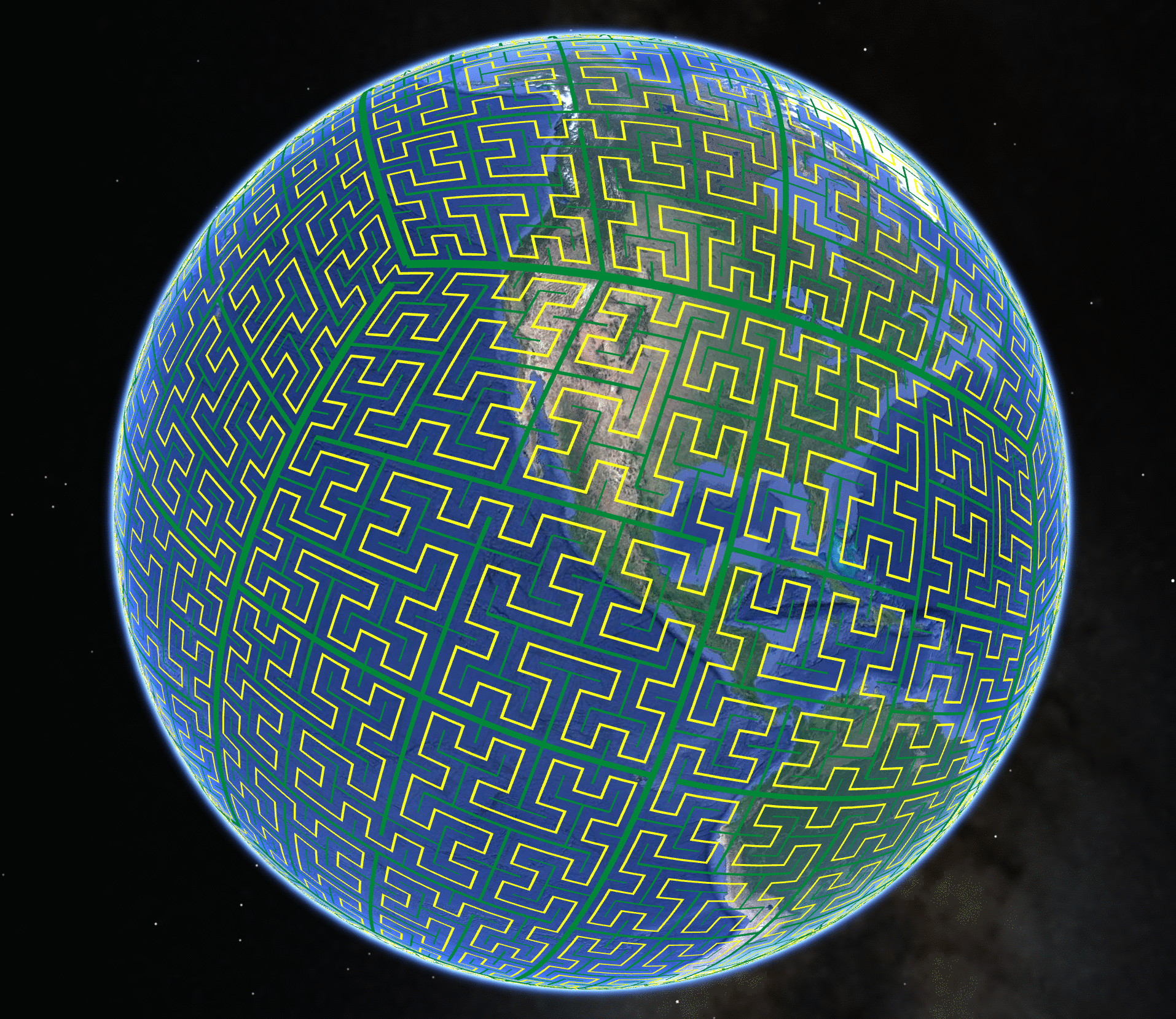``````

ijToPos = int{
{0, 1, 3, 2}, // canonical order
{0, 3, 1, 2}, // axes swapped
{2, 3, 1, 0}, // bits inverted
{2, 1, 3, 0}, // swapped & inverted
}
posToIJ = int{
{0, 1, 3, 2}, // canonical order:    (0,0), (0,1), (1,1), (1,0)
{0, 2, 3, 1}, // axes swapped:       (0,0), (1,0), (1,1), (0,1)
{3, 2, 0, 1}, // bits inverted:      (1,1), (1,0), (0,0), (0,1)
{3, 1, 0, 2}, // swapped & inverted: (1,1), (0,1), (0,0), (1,0)
}

``````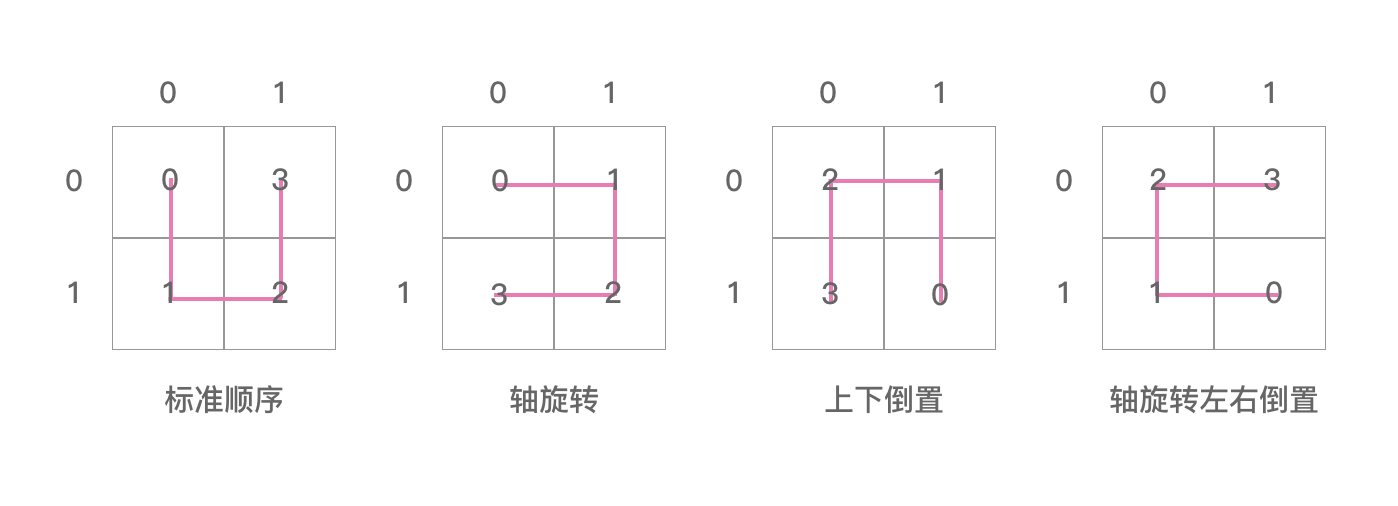posToIJ 的四张图其实是“ U ” 字形逆时针分别旋转90°得到的。这里我们只能看出四张图相互之间的联系，即兄弟之间的联系，但是看不到父子图相互之间的联系。

posToIJ = {0, 1, 3, 2} 里面存的值是 ij 合在一起表示的值。posToIJ = 0，指的是 i = 0，j = 0 的那个方格，ij 合在一起是00，即0。posToIJ = 1，指的是 i = 0，j = 1 的那个方格，ij 合在一起是01，即1。posToIJ = 1，指的是 i = 1，j = 1 的那个方格，ij 合在一起是11，即3。posToIJ = 2，指的是 i = 1，j = 0 的那个方格，ij 合在一起是10，即2。数组里面的顺序是 “ U ” 字形画的顺序。所以 posToIJ = {0, 1, 3, 2} 表示的是图0中的样子。其他图形同理。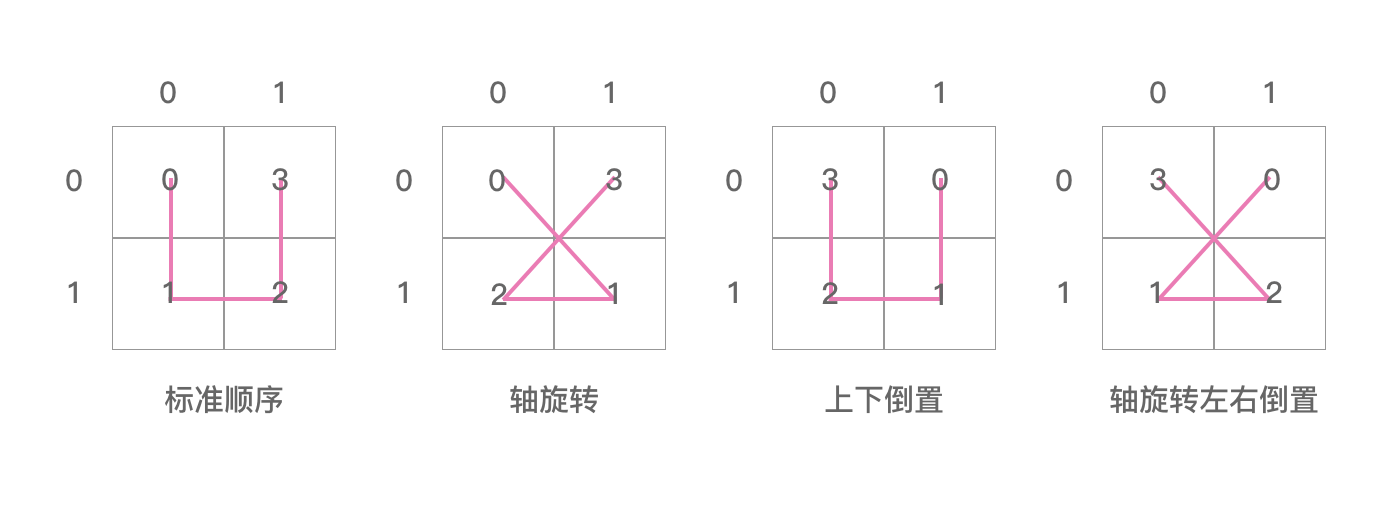``````
func init() {
initLookupCell(0, 0, 0, 0, 0, 0)
}

``````

``````
func init() {
initLookupCell(0, 0, 0, 0, 0, 0)
initLookupCell(0, 0, 0, 1, 0, 1)
initLookupCell(0, 0, 0, 2, 0, 2)
initLookupCell(0, 0, 0, 3, 0, 3)
}

``````

initLookupCell 入参有6个参数，有4个参数都是0，我们需要重点关注的是第四个参数和第六个参数。第四个参数是 origOrientation，第六个参数是 orientation。

``````
initLookupCell(level, i+(r>>1), j+(r&1), origOrientation, pos, orientation^posToOrientation)
initLookupCell(level, i+(r>>1), j+(r&1), origOrientation, pos+1, orientation^posToOrientation)
initLookupCell(level, i+(r>>1), j+(r&1), origOrientation, pos+2, orientation^posToOrientation)
initLookupCell(level, i+(r>>1), j+(r&1), origOrientation, pos+3, orientation^posToOrientation)

``````

``````
r := posToIJ[orientation]

``````

r 数组来自于 posToIJ 数组。posToIJ 数组上面说过了，它里面装的其实是4个不同方向的“ U ”字。相当于表示了当前四个小方格兄弟相互之间的方向。r、r、r、r 取出的其实就是 00，01，10，11 这4个数。那么 r>>1 操作就是取出二位二进制位的前一位，即 i 位。r&1 操作就是取出二位二进制位的后一位，即 j 位。r、r、r 同理。

``````
orientation^posToOrientation
orientation^posToOrientation
orientation^posToOrientation
orientation^posToOrientation

``````

``````

``````

``````
posToOrientation = int{1, 0, 0, 3}

``````

posToOrientation 数组里面装的原始的值是 [01，00，00，11]，这个4个数值并不是随便初始化的。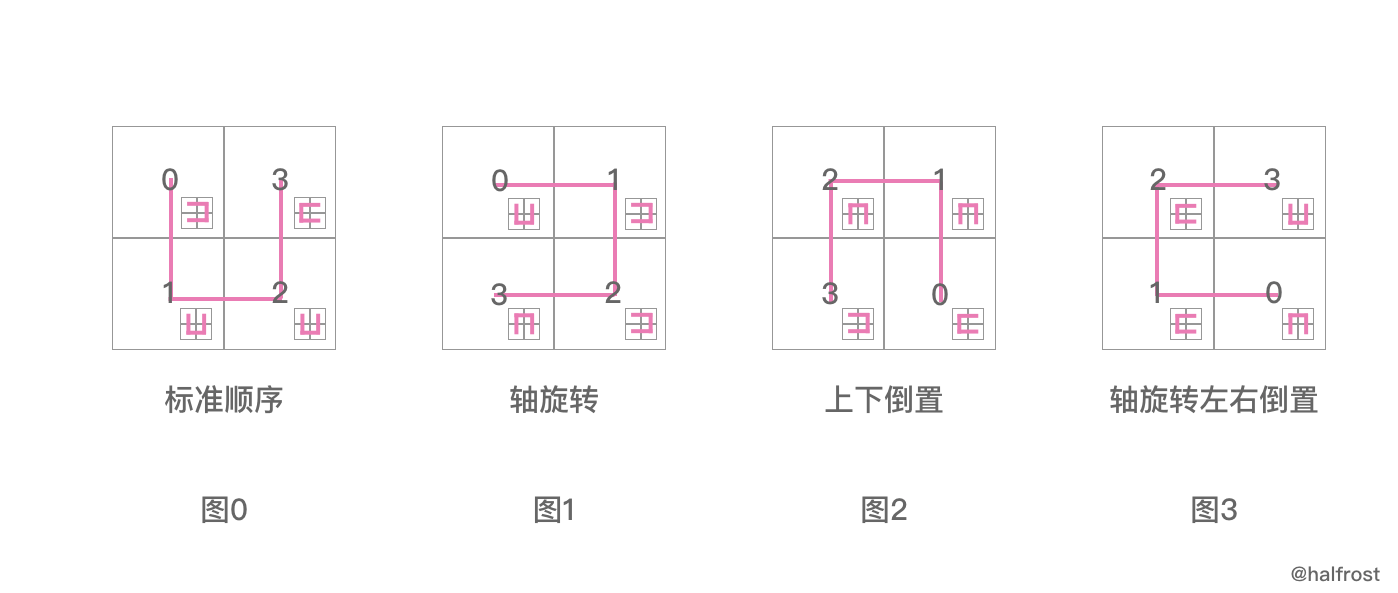posToIJ 的四张图我们只能看出兄弟之间的关系，那么 posToOrientation 的四张图让我们知道了父子之间的关系。

``````
orientation^posToOrientation
orientation^posToOrientation
orientation^posToOrientation
orientation^posToOrientation

````````````
orientation^posToOrientation
orientation^posToOrientation
orientation^posToOrientation
orientation^posToOrientation

``````

``````
00 ^ 01 = 01
00 ^ 00 = 00
00 ^ 00 = 00
00 ^ 11 = 11

``````

orientation = 1，orientation = 2，orientation = 3，都是同理的：

``````
01 ^ 01 = 00
01 ^ 00 = 01
01 ^ 00 = 01
01 ^ 11 = 10

10 ^ 01 = 11
10 ^ 00 = 10
10 ^ 00 = 10
10 ^ 11 = 01

11 ^ 01 = 10
11 ^ 00 = 11
11 ^ 00 = 11
11 ^ 11 = 00

``````

``````
lookupPos[(ij<<2)+origOrientation] = (pos << 2) + orientation
lookupIJ[(pos<<2)+origOrientation] = (ij << 2) + orientation

``````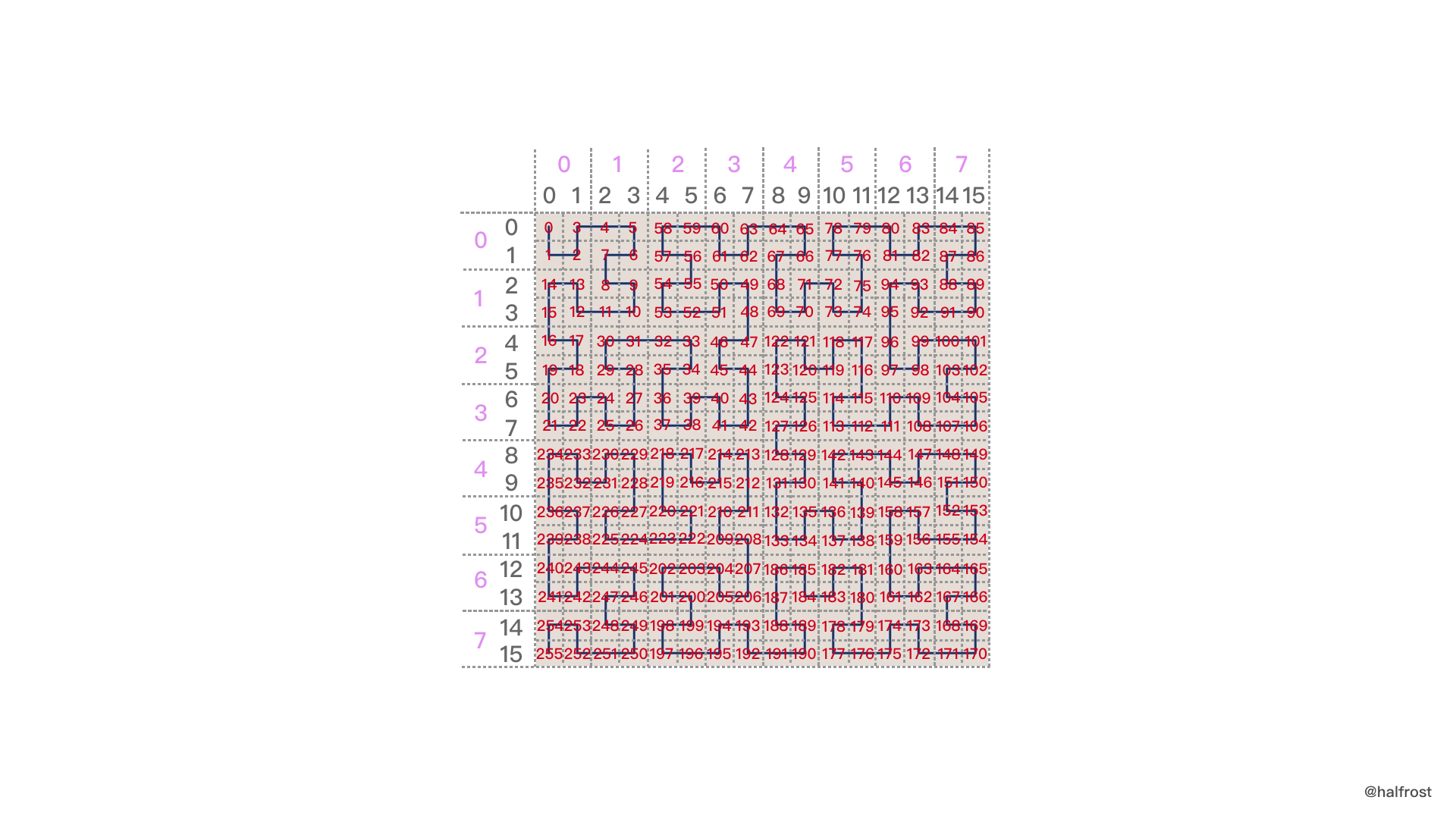pos 指的是在 希尔伯特曲线上的位置。这个位置是从 希尔伯特 曲线的起点开始算的。从起点开始数，到当前是第几块方块。注意这个方块是由 4 个小方块组成的大方块。因为 orientation 是选择4个方块中的哪一个。

i，j 并不是直接对应的 希尔伯特曲线 坐标系上的坐标。因为初始化需要生成的是五阶希尔伯特曲线。在 posToIJ 数组表示的一阶希尔伯特曲线，所以 i，j 才直接对应的 希尔伯特曲线 坐标系上的坐标。

pos 参数对应的就是希尔伯特曲线坐标系上的坐标。一旦一个希尔伯特曲线的起始点和阶数确定以后，四个小方块组成的一个大方块的 pos 位置确定以后，那么它的坐标其实就已经确定了。希尔伯特曲线上的坐标并不依赖 i，j，完全是由曲线的性质和 pos 位置决定的。

i，j 对应的是一个经过坐标变换以后的坐标系坐标。

i，j 和 pos 互相转换之间的桥梁就是生成希尔伯特曲线的方式。这种方式可以类比 Z - index 曲线的生成方式。

Z - index 曲线的生成方式是把经纬度坐标分别进行区间二分，在左区间的记为0，在右区间的记为1 。将这两串二进制字符串偶数位放经度，奇数位放纬度，最终组合成新的二进制串，这个串再经过 base-32 编码以后，最终就生成了 geohash 。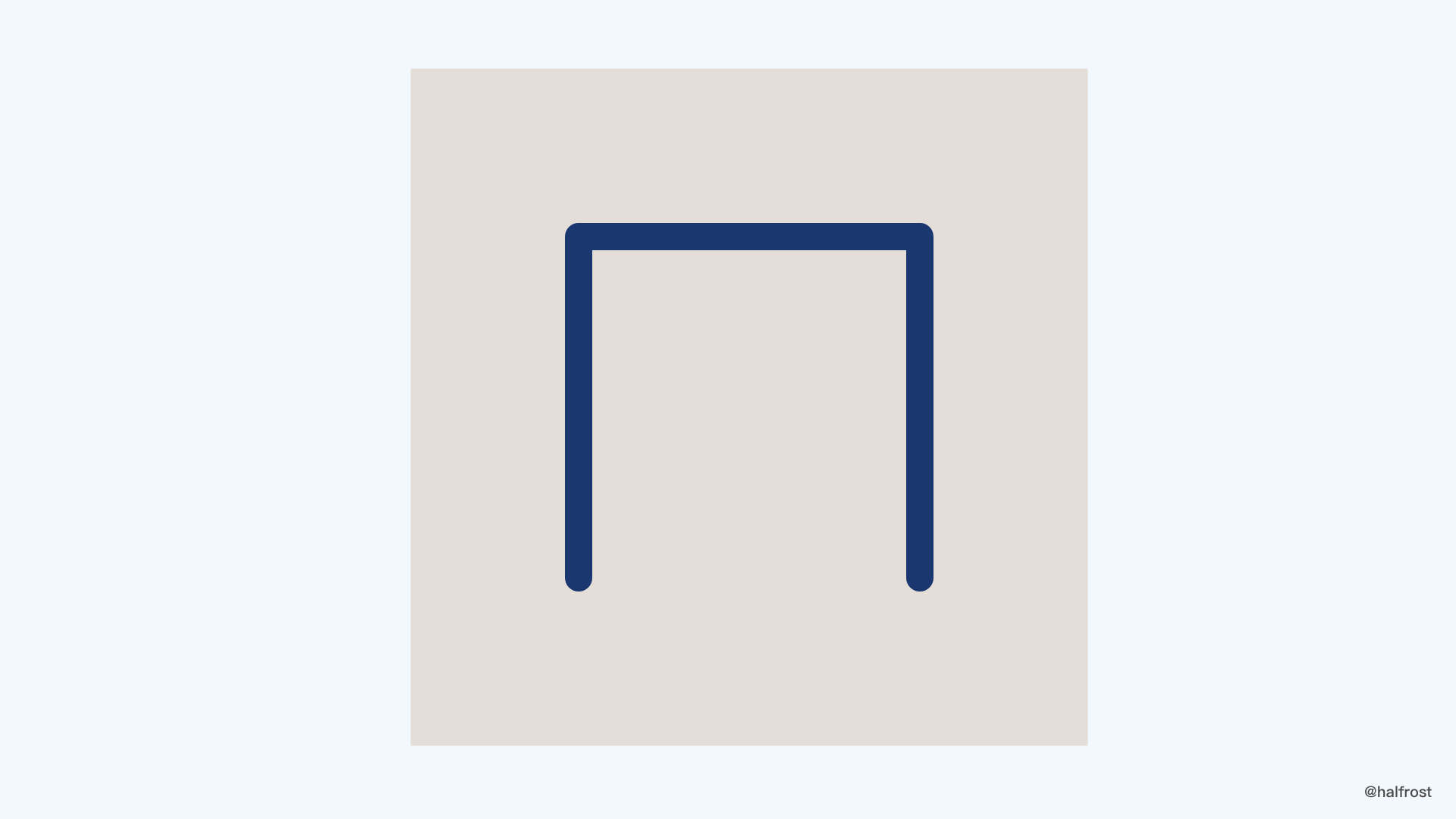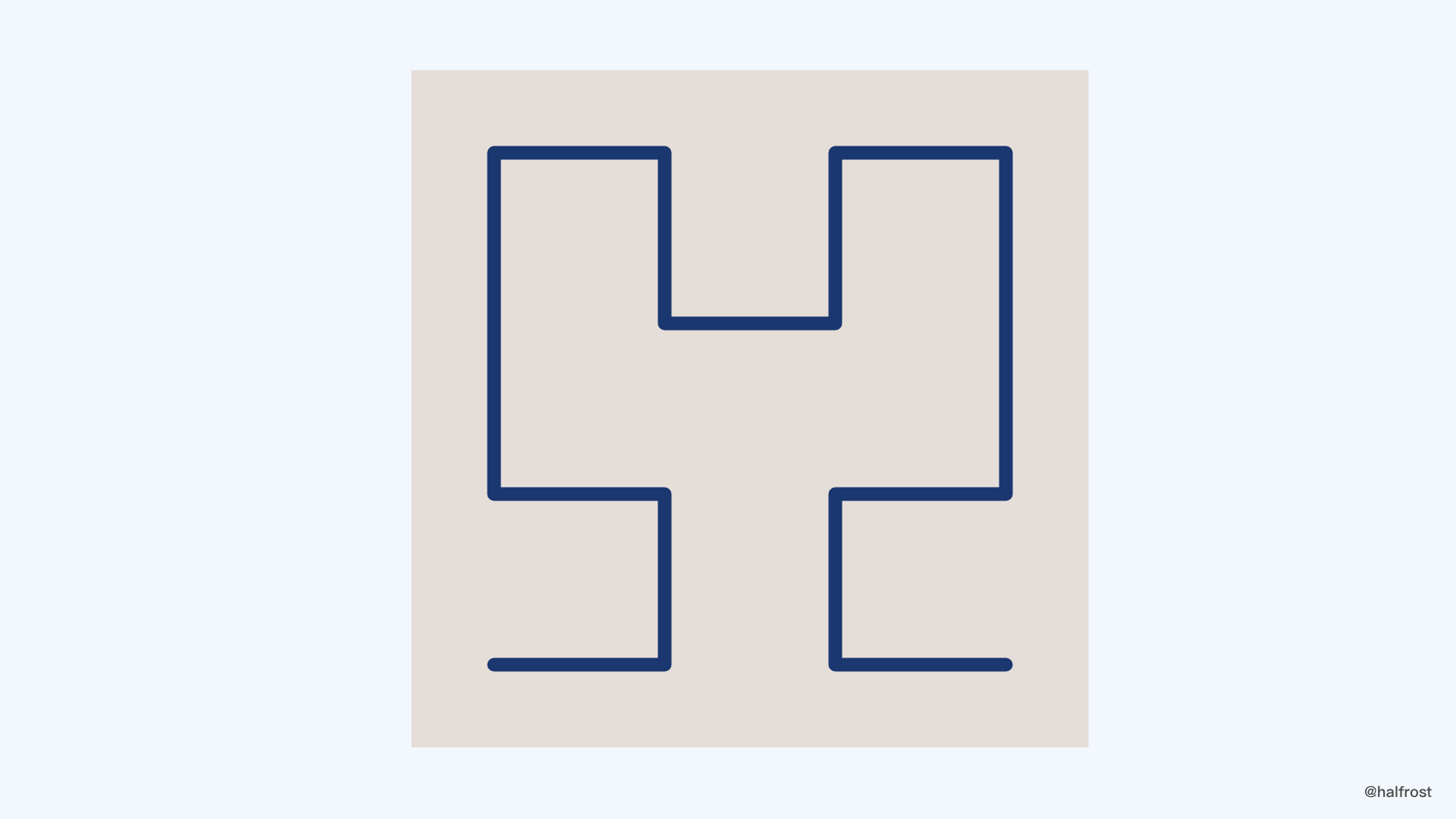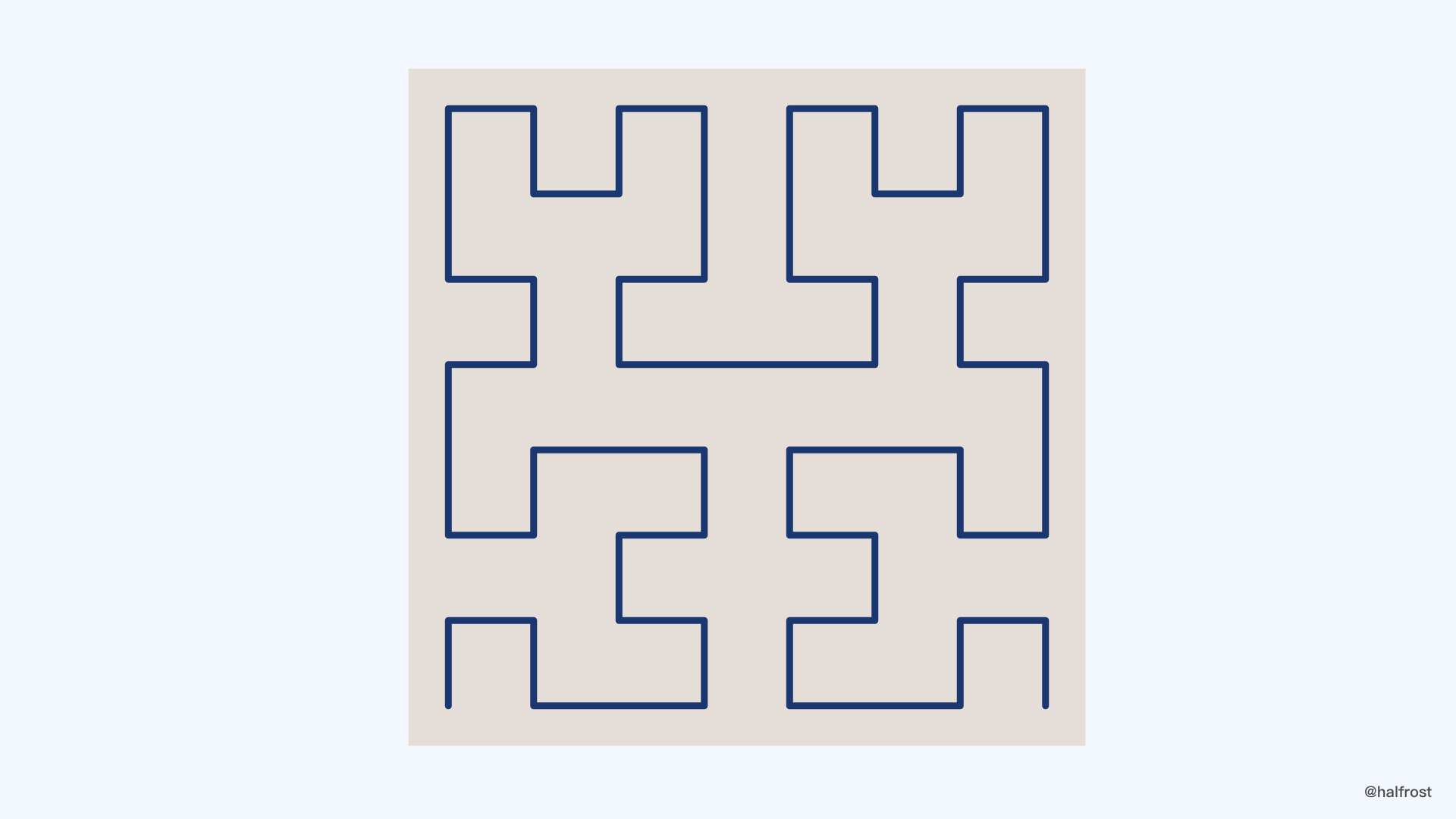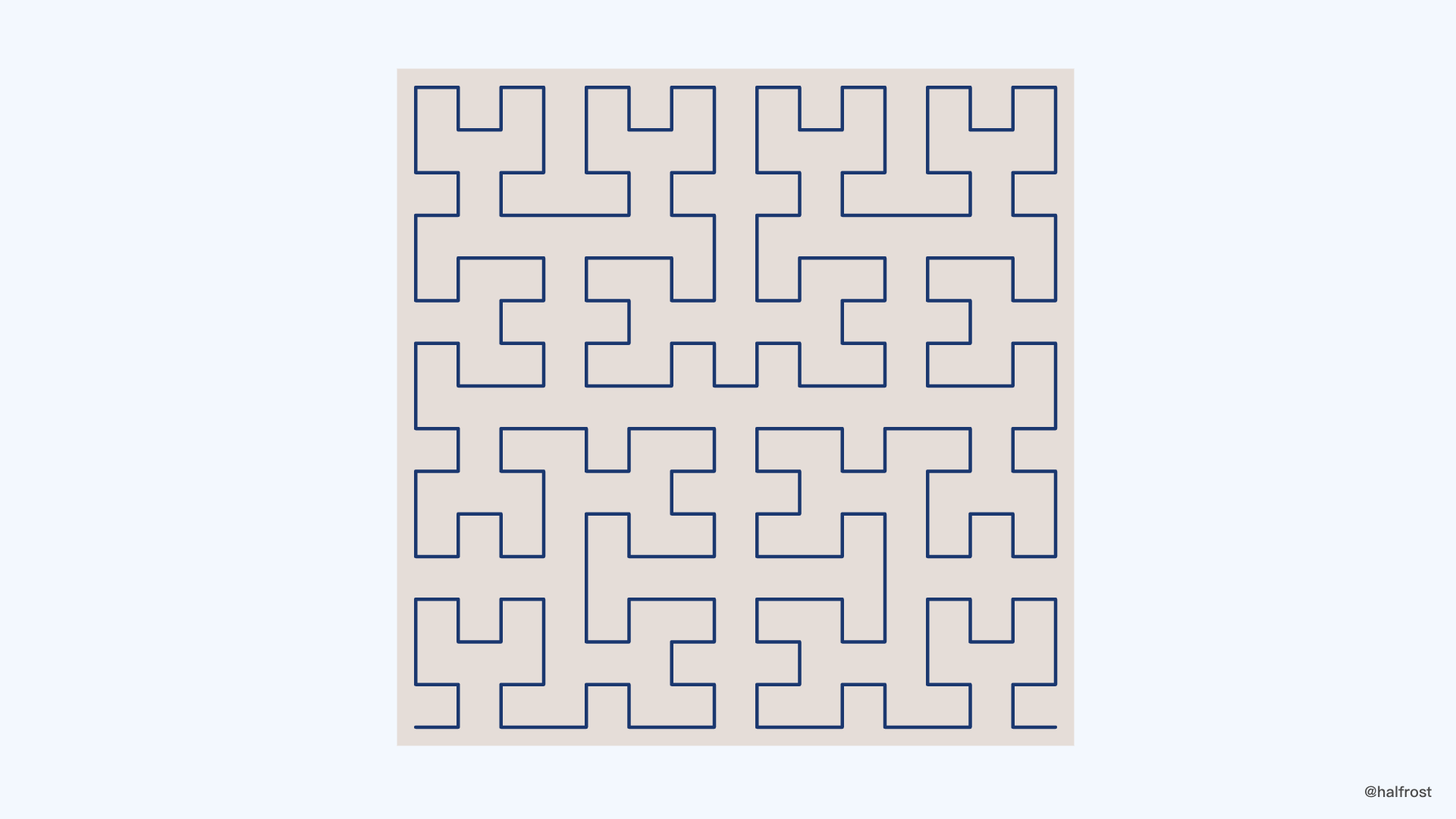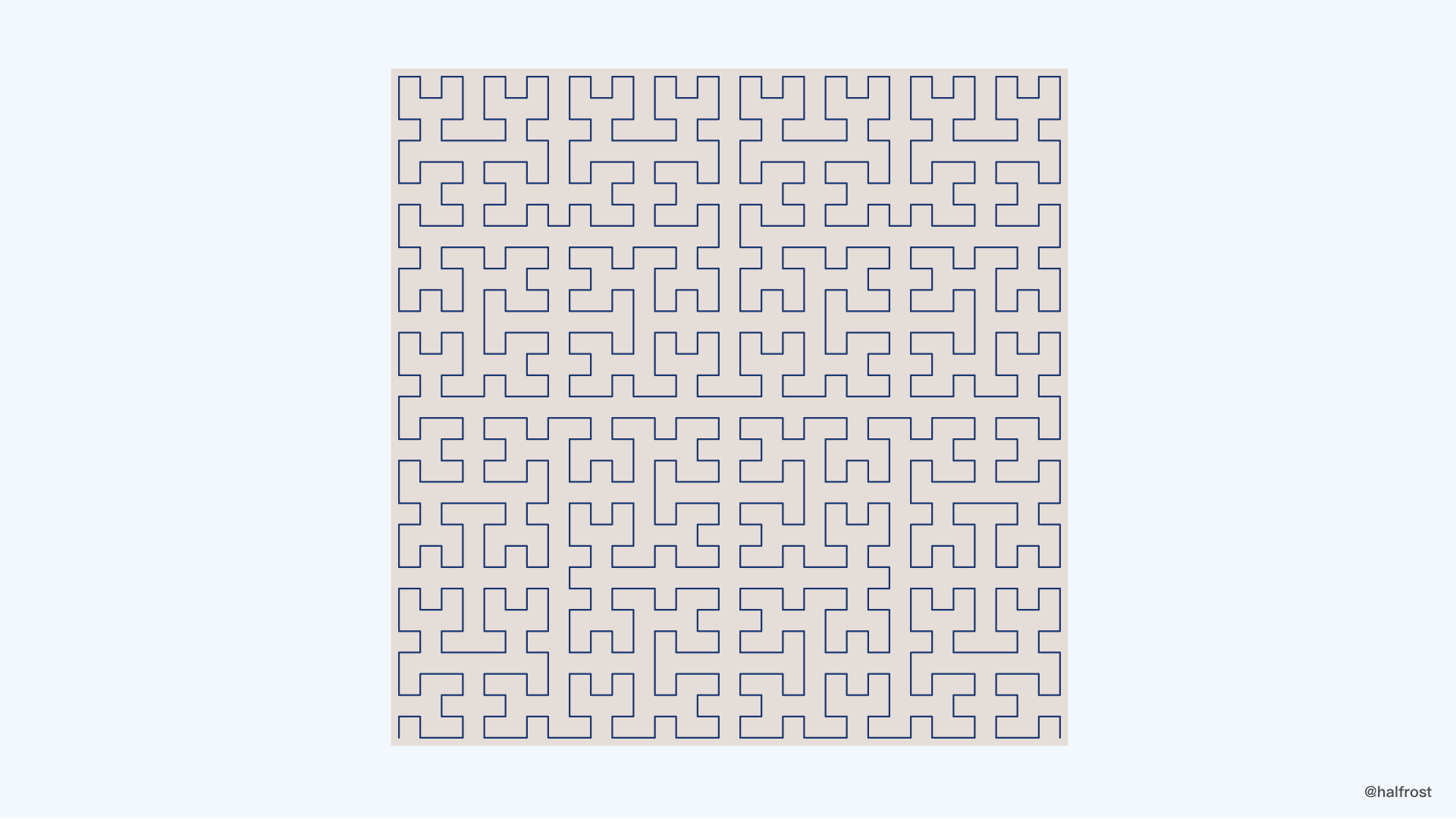## 七. 举例

``````
func cellIDFromFaceIJ(f, i, j int) CellID {
// 1.
n := uint64(f) << (posBits - 1)
// 2.
bits := f & swapMask
// 3.
for k := 7; k >= 0; k-- {
mask := (1 << lookupBits) - 1
bits += int((i>>uint(k*lookupBits))&mask) << (lookupBits + 2)
bits += int((j>>uint(k*lookupBits))&mask) << 2
bits = lookupPos[bits]
// 4.
n |= uint64(bits>>2) << (uint(k) * 2 * lookupBits)
// 5.
}
// 6.
return CellID(n*2 + 1)
}

``````

1. 将 face 左移 60 位。
2. 计算初始的 origOrientation。初始的 origOrientation 是 face 转换得来的，face & 01 以后的结果是为了使每个面都有一个右手坐标系。
3. 循环，从头开始依次取出 i ，j 的4位二进制位，计算出 ij<<2 + origOrientation，然后查 lookupPos 数组找到对应的 pos<<2 + orientation 。
4. 拼接 CellID，右移 pos<<2 + orientation 2位，只留下 pos ，把pos 继续拼接到 上次循环的 CellID 后面。
5. 计算下一个循环的 origOrientation。&= (swapMask | invertMask) 即 & 11，也就是取出末尾的2位二进制位。
6. 最后拼接上最后一个标志位 1 。

``````
000 & 001 = 00
001 & 001 = 01
010 & 001 = 00
011 & 001 = 01
100 & 001 = 00
101 & 001 = 01

``````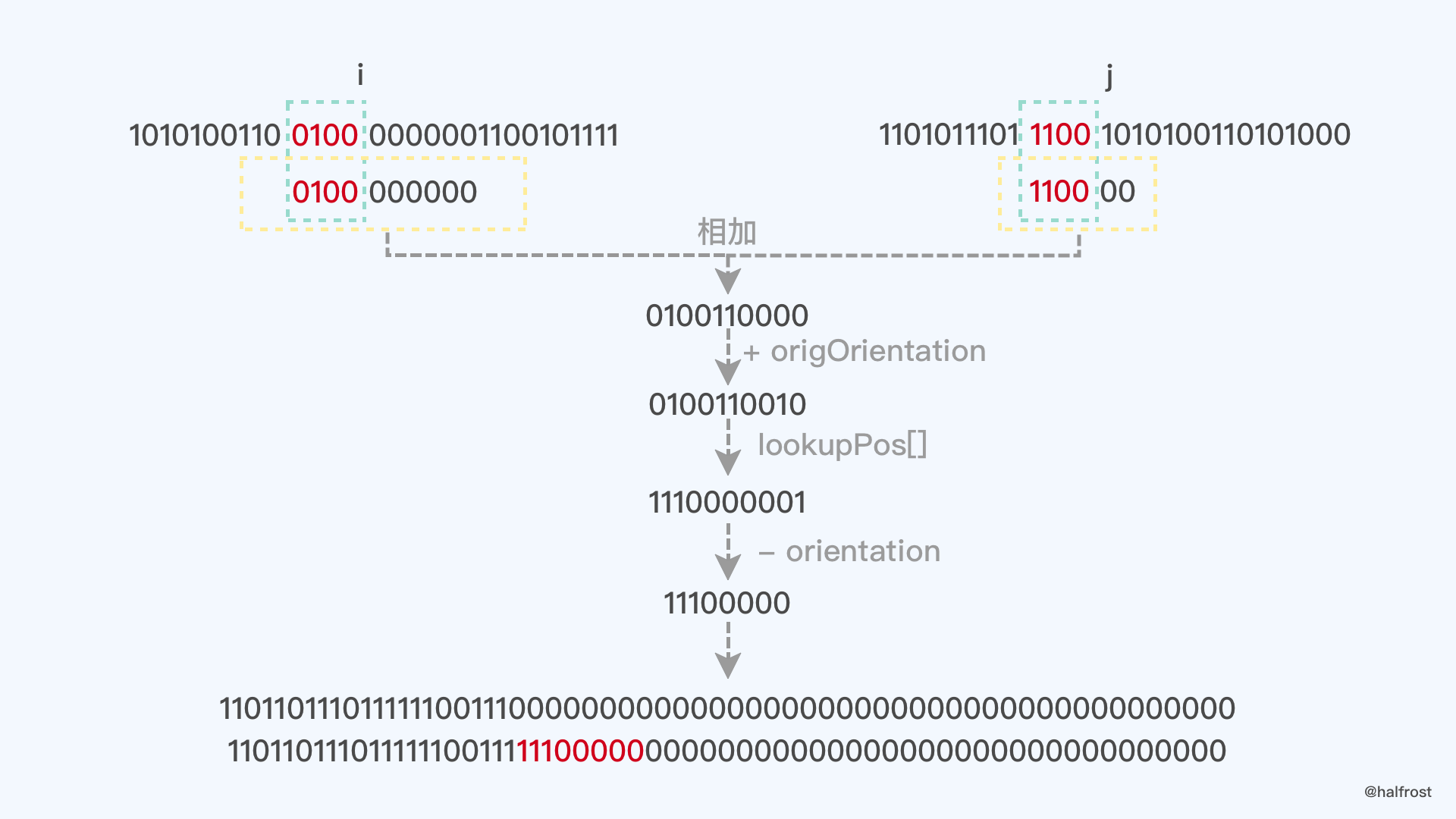GitHub Repo：Halfrost-Field

Follow: halfrost · GitHubPrevious Post

Next Post